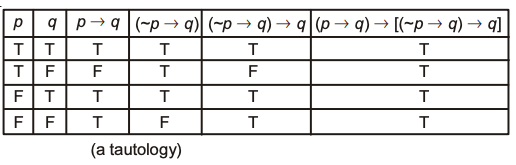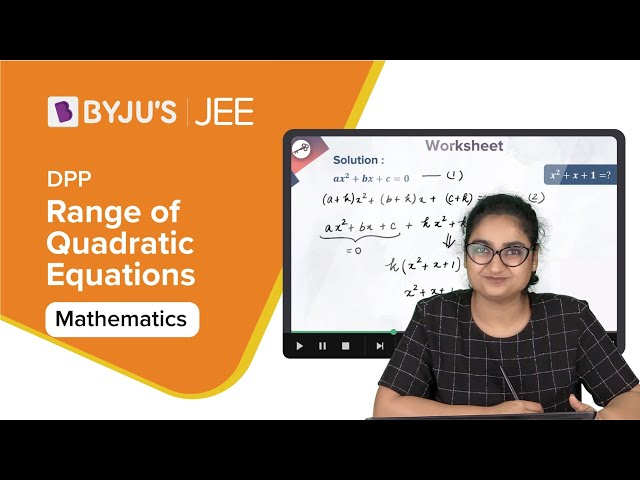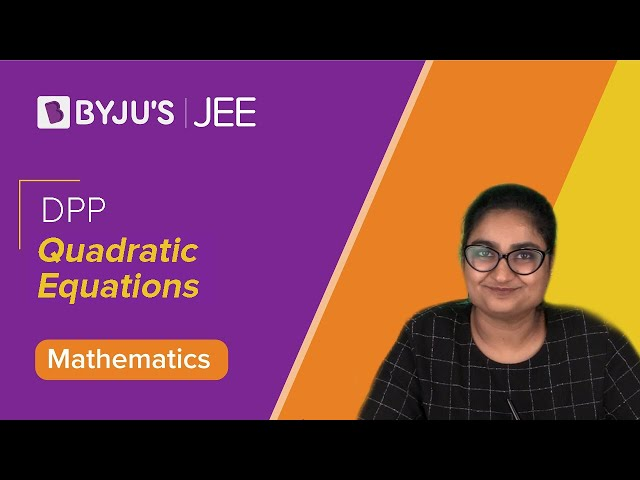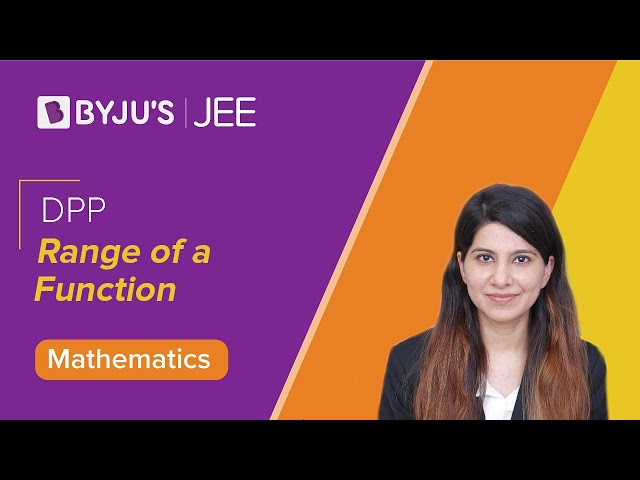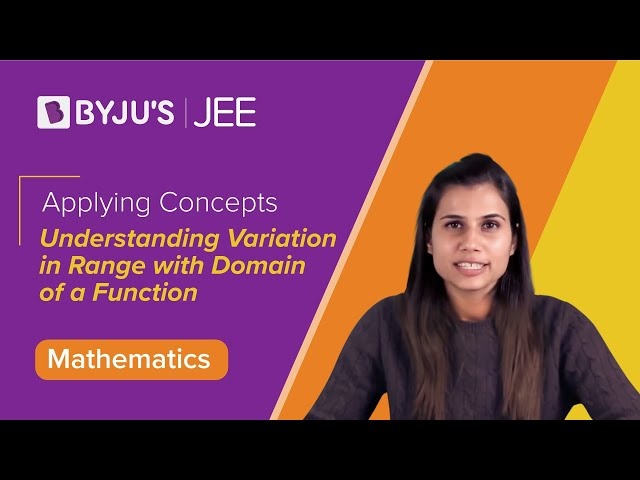Win up to 100% scholarship on Aakash BYJU'S JEE/NEET courses with ABNAT Win up to 100% scholarship on Aakash BYJU'S JEE/NEET courses with ABNAT

# JEE Main 2017 Maths Paper With Solutions (April 2)

JEE Main 2017 Paper with Solutions Maths – Offline Exam will help the students to get familiar with the difficulty level of the questions, which can be asked in the upcoming JEE Main examination. The students appearing for the examination are required to solve the question paper to check their preparedness. The solutions uploaded at BYJU’S will enable the students to identify the important topics. Practising these questions will help them revisit and update their revision strategies.
April 2nd – Maths

Question 1: The function f : R → [(-1/2), (1/2)] is defined as f(x) = [x]/[1 + x2], is:

1) injective but not surjective.
2) surjective but not injective.
3) neither injective nor surjective.
4) invertible.

f(x) = [x]/[1 + x2]

$$\begin{array}{l}f'(x)=\frac{(1+x^{2})*1-x*2x}{(1+x^{2})^{2}}\\ =\frac{1-x^{2}}{(1+x^{2})^{2}}\end{array}$$

∴ Not injective.

y = (x / (1 + x2))

yx2 – x + y = 0

For y ≠ 0

D = 1 – 4y2 ≥ 0

y [(-1 / 2), (1 / 2)] – {0}

For, y = 0 ⇒ x = 0

∴Part of range

∴Range = [(-1 / 2), (1 / 2)]

∴Surjective but not injective.

Question 2: If, for a positive integer n, the quadratic equation,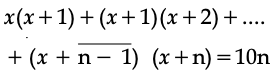has two consecutive integral solutions, then n is equal to:

1) 9
2) 10
3) 11
4) 12

Rearranging equation, we get

nx2 + {1 + 3 + 5 + ….. + (2n – 1)x + {1.2 + 2.3 + ….. + (n – 1)n} = 10n

nx2 + n2x + [(n – 1) n (n + 1)] / 3 = 10n

x2 + nx + [n2 – 31] / 3 = 0

Given difference of roots = 1

|ɑ – β| = 1

D = 1

n2 – (4 / 3) (n2 – 31) = 1

n = 11

Question 3: Let ω be a complex number such that 2ω + 1 = z where z = √-3. If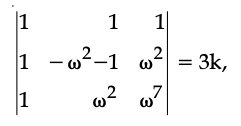then k is equal to,

1) z
2) −1
3) 1
4) − z

2ω + 1 = z, z = √3i

ω = (-1 + √3i) / 2 [Cube root of unity]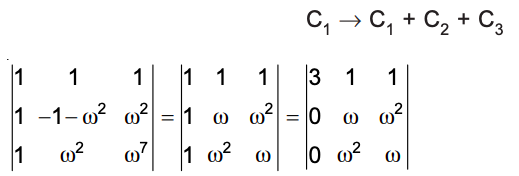= 3 (ω2 – ω4)

= 3 [{(-1 – √3i) / 2} – {(-1 + √3i) / 2}]

= – 3√3i

= -3z

k = -z

Question 4: If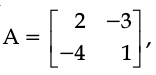then adj (3A2 + 12A) is equal to :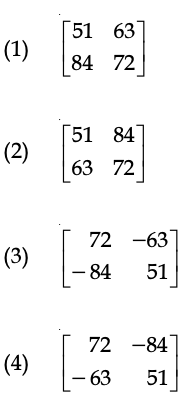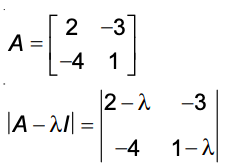= (2 – 2λ – λ + λ2) – 12

f (λ) = λ2 – 3λ – 10

∵ A satisfies f (λ).

A2 – 3A – 10I = 0

A2 – 3A = 10I

3A2 – 9A = 30I

3A2 + 12A = 30I + 21A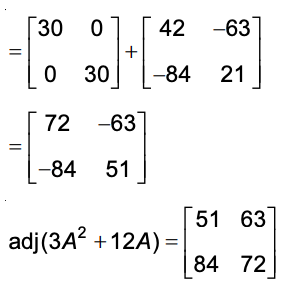Question 5: If S is the set of distinct values of ‘b’ for which the following system of linear equations

x + y + z = 1

x + ay + z = 1

ax + by + z = 0

has no solution, then S is :

1) an infinite set
2) a finite set containing two or more elements
3) a singleton
4) an empty set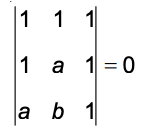⇒ – (1 – a2) = 0

⇒ a = 1

For a = 1

Eq. (1) & (2) are identical i.e.,x + y + z = 1.

To have no solution with x + by + z = 0.

b = 1

Question 6: A man X has 7 friends, 4 of them are ladies and 3 are men. His wife Y also has 7 friends, 3 of them are ladies and 4 are men. Assume X and Y have no common friends. Then the total number of ways in which X and Y together can throw a party inviting 3 ladies and 3 men, so that 3 friends of each of X and Y are in this party, is :

1) 468
2) 469
3) 484
4) 485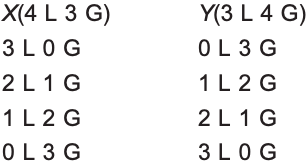Required number of ways

= 4C3 . 4C3 + (4C2 . 3C1)2 + (4C1 . 3C2)2 + (3C3)2

= 16 + 324 + 144 + 1

= 485

Question 7: The value of (21C110C1) + (21C210C2) + (21C310C3) + (21C410C4) + … (21C1010C10) is

1) 221 − 210
2) 220 − 29
3) 220 − 210
4) 221 − 211

21C1 + 21C2 + …… 21C10 = (1 / 2) (21C0 + 21C1 + ….. 21C21) – 1

= 220 – 1

10C1 + 10C2 + …. 10C10 = 210 – 1

Required sum = (220 – 1) – (210 – 1)

= 220 – 210

Question 8: For any three positive real numbers a, b and c, 9 (25a2 + b2) + 25 (c2 − 3ac) = 15b (3a + c). Then :

1) b, c and a are in A.P.
2) a, b and c are in A.P.
3) a, b and c are in G.P.
4) b, c and a are in G.P.

9 (25a2 + b2) + 25 (c2 − 3ac) = 15b (3a + c)

⇒ (15a)2 + (3b)2 + (5c)2 – 45b – 15b – 75ac = 0

(15a – 3b)2 + (3b – 5c)2 + (15a – 5c)2 = 0

It is possible when

15a – 3b = 0 and 3b – 5c = 0 and 15a – 5c = 0

15a = 3b = 5c

(a / 1) = (b / 5) = (c / 3)

∴ b, c, a are in A.P.

Question 9: Let a, b, c R. If f (x) = ax2 + bx + c is such that a + b + c = 3 and f (x + y) = f (x) + f (y) + xy, ∀ x, y R, then

$$\begin{array}{l}\sum_{n=1}^{10}\end{array}$$
f (n) = is equal to :

1) 165
2) 190
3) 255
4) 330

As f (x + y) = f (x) + f (y) + xy

Given, f (1) = 3

Putting, x = y = 1

⇒ f (2) = 2 f (1) + 1 = 7

Similarly, x = 1, y = 2,

⇒ f (3) = f (1) + f (2) + 2 = 12

Now,

$$\begin{array}{l}\sum_{n=1}^{10}\end{array}$$
f (n) = f (1) + f (2) + …. f (10)

= 3 + 7 + 12 + 18 + …

= S (let)

Now, Sn = 3 + 7 + 12 + 18 … + tn

Again, Sn = 3 + 7 + 12 + 18 … + tn-1 + tn

We get, tn = 3 + 4 + 5 + …. n terms

= [n (n + 5)] / 2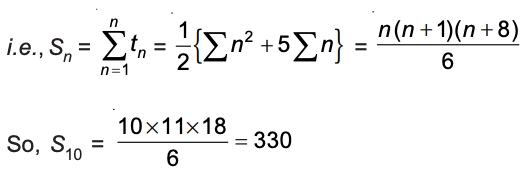Question 10:

$$\begin{array}{l}\lim_{x\rightarrow \pi/2}\frac{cotx-cosx}{(\pi-2x)^{3}}\end{array}$$
equals:

1) 1 / 16
2) 1 / 8
3) 1 / 4
4) 1 / 24

$$\begin{array}{l}\lim_{x\rightarrow \pi/2}\frac{cotx-cosx}{(\pi-2x)^{3}}\\ \text \ Put \ x=\frac{\pi}{2}-x=t\\ =\lim_{t=0}\frac{tant-sint}{8t^{3}}\\ =\lim_{t=0}\frac{sint*2sin^{2}\frac{t}{2}}{8t^{3}}\\ =\frac{1}{16}\end{array}$$

Question 11: If for x ∈ (0, (1 / 4)), the derivative of tan-1 (6x√x / (1 – 9x3)) is √x . g(x), then g(x) equals:

1) (3x√x / (1 – 9x3))
2) (3x/(1 – 9x3))
3) (3/(1 + 9x3))
4) (9/(1 + 9x3))

f (x) = 2 tan-1 (3x√x) for x ∈ (0, (1/4))

f ‘ (x) = (9√x/(1 + 9x3))

g (x) = (9/(1 + 9x3))

Question 12: The normal to the curve y (x − 2) (x − 3) = x + 6 at the point where the curve intersects the y-axis passes through the point :

1) (1/2, 1/2)
2) (1/2, -1/3)
3) (1/2, 1/3)
4) (-1/2, -1/2)

y (x – 2) (x – 3) = x + 6

At y-axis, x = 0, y = 1

Now, on differentiation,

(dy / dx) (x – 2) (x – 3) + y (2x – 5) = 1

(dy / dx) (6) + 1 * (-5) = 1

(dy / dx) = 6 / 6 = 1

Now slope of normal = –1

Equation of normal y – 1 = –1 (x – 0)

y + x – 1 = 0 … (i)

Line (i) passes through (1 / 2, 1 / 2).

Question 13: Twenty meters of wire is available for fencing off a flower-bed in the form of a circular sector. Then the maximum area (in sq. m) of the flower-bed, is :

1) 10
2) 25
3) 30
4) 12.52r + θr = 20 —- (i)

A = Area = (θ / 2π) * (πr2) = (θr2 / 2) —- (ii)

A = (r2 / 2) ([20 – 2r] / r)

A = [(20r – 2r2) / 2] = 10r – r2

A to be maximum

dA / dr = 10 – 2r = 0 ⇒ r = 5

d2A / dr2 = -2 < 0

Hence for r = 5, A is maximum

Now, 10 + (θ * 5) = 20 ⇒ θ = 2 (radian)

Area = (2 / 2π) * (π) (5)2 = 25 sq m

Question 14: Let In = ∫tann x dx, (n > 1). If I4 + I6 = a tan5 x + bx5 + C, where C is a constant of integration, then the ordered pair (a, b) is equal to :

1) (1/5, 0)
2) (1/5, -1)
3) (-1/5, 0)
4) (-1/5, 1)

In = ∫tann x dx, (n > 1)

I4 + I6 = ∫(tan4 x + tan6 x) dx

= ∫tan4 x sec2 x dx

Let tan x = t

sec2 x dx = dt

= ∫t4 dt

= (t5/5) + C

= (1/5) tan5 x + C

a = (1/5), b = 0

Question 15: The integral

$$\begin{array}{l}\int_{\frac{\pi}{4}}^{\frac{3\pi}{4}}\frac{dx}{1+cosx}\end{array}$$
is equal to:

1) 2
2) 4
3) −1
4) −2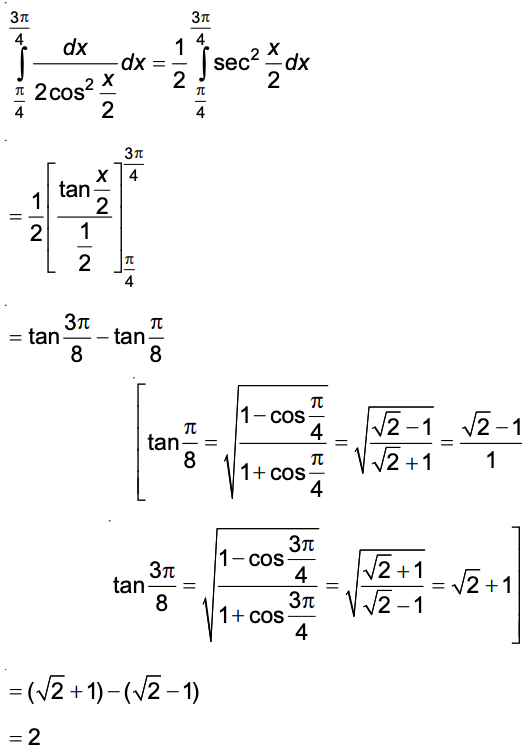Question 16: The area (in sq. units) of the region {(x, y) : x ≥ 0, x + y ≤ 3, x2 ≤ 4y and y ≤ 1 + x} is :

1) 3 / 2
2) 7 / 3
3) 5 / 2
4) 59 / 12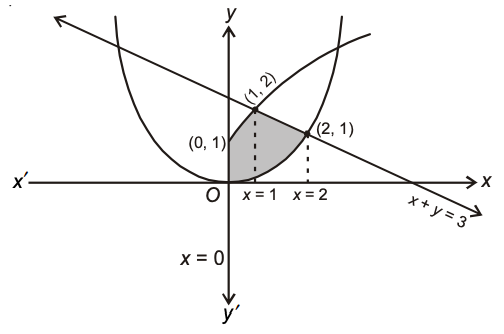=

$$\begin{array}{l}\int_{0}^{1}(\sqrt{x}+1-\frac{x^{2}}{4})dx+\int_{1}^{2}((3-x)-\frac{x^{2}}{4})dx\\ =\frac{5}{2}\end{array}$$
sq units

Question 17: If (2 + sinx) (dy/dx) + (y + 1) cosx = 0 and y(0) = 1, then y(π/2) is equal to:

1) -2/3
2) -1/3
3) 4/3
4) 1/3

(2 + sinx) (dy/dx) + (y + 1) cos x = 0

y(0) = 1, y(π / 2) = ?

(1/(y + 1)) dy + (cos x/[2 + sinx]) dx = 0

ln |y + 1| + ln (2 + sinx) = ln C

(y + 1) (2 + sinx) = C

Put x = 0, y = 1

(1 + 1) . 2 = C ⇒ C = 4

Now, (y + 1) (2 + sinx) = 4

(y + 1) = 4 / 3

y = (4 / 3) – 1 = 1 / 3

Question 18: Let k be an integer such that the triangle with vertices (k, −3k), (5, k) and (−k, 2) has area 28 sq. units. Then the orthocentre of this triangle is at the point :

1) (1, 3/4)
2) (1, -3/4)
3) (2, 1/2)
4) (2, -1/2)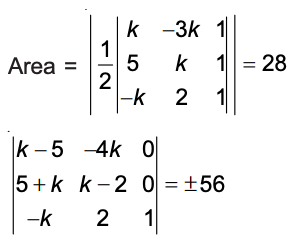(k2 – 7k + 10) + 4k2 + 20k = ± 56

5k2 + 13k – 46 = 0

5k2 + 13k + 66 = 0

5k2 + 13k – 46 = 0

k = [-13 ± √169 + 920]/10

= 2, – 4.6 [reject]

For k = 2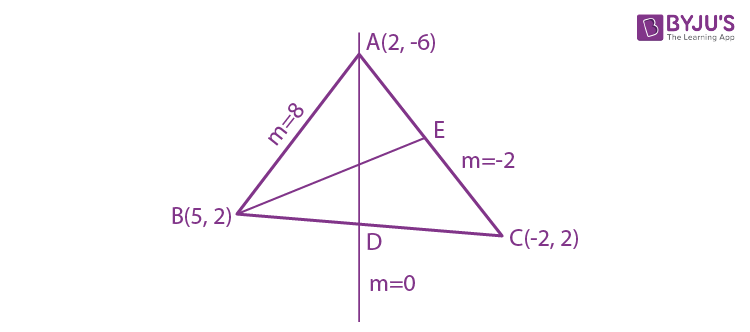x = 2 …(i)

Also, equation of BE,

(y – 2) = (1/2) (x – 5)

2y – 4 = x – 5

x – 2y – 1 = 0 …(ii)

Solving (i) & (ii), 2y = 1

y = 1 / 2

Orthocentre is (2, 1/2)

Question 19: The radius of a circle, having minimum area, which touches the curve y = 4 − x2 and the lines, y = |x| is :

1) 2 (√2 – 1)
2) 4 (√2 – 1)
3) 4 (√2 + 1)
4) 2 (√2 + 1)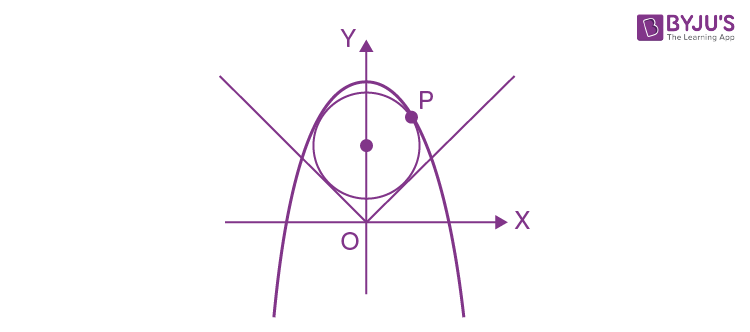x2 = – (y – 4)

Let a point on the parabola P [(t/2) , (4 – (t2/4))]

Equation of normal at P is

y + (t2 / 4) – 4 = (1 / t) (x – [t / 2])

x – ty – (t3 / 4) + (7 / 2)t = 0

It passes through centre of circle, say (0, k)

-tk – (t3 / 4) + (7 / 2)t = 0 —- (i)

t = 0, t2 = 14 – 4k

Radius = r = |(0 – k) / √2| (Length of perpendicular from (0, k) to y = x)

r = k / √2

Equation of circle is x2 + (y – k)2 = k2 / 2

It passes through point P

(t2 / 4) + [4 – (t2 / 4) – k]2 = k2 / 2

t4 + t2 [8k – 28] + 8k2 – 128k + 256 = 0 —- (ii)

For t = 0 ⇒ k2 – 16k + 32 = 0

k = 8 ± 4√2

r = k / √2 = 4 (√2 – 1) (discarding 4 (√2 + 1)) —– (iii)

For t = ± √(14 – 4k)

(14 – 4k)2 + (14 – 4k) (8k – 28) + 8k2 – 128k + 256 = 0

2k2 + 4k – 15 = 0

k = [-2 ± √34] /

r = k / √2 = [√17 – √2] / 2 (Ignoring negative value of r) ..(iv)

From (iii) & (iv),

rmin = [√17 – √2] / 2

But from options, 4 (√2 – 1)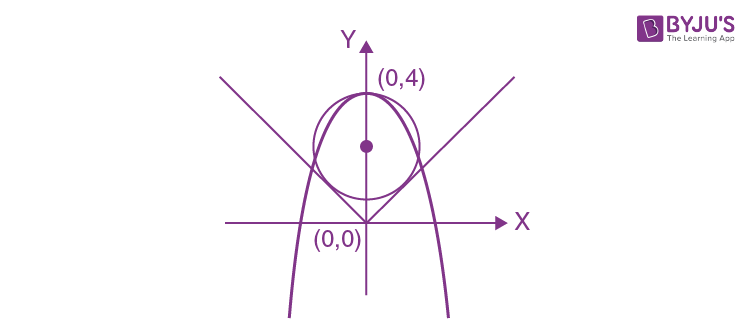Question 20: The eccentricity of an ellipse whose centre is at the origin is 1 / 2. If one of its directrices is x = −4, then the equation of the normal to it at (1, (3 / 2)) is:

1) 4x − 2y = 1
2) 4x + 2y = 7
3) x + 2y = 4
4) 2y − x = 2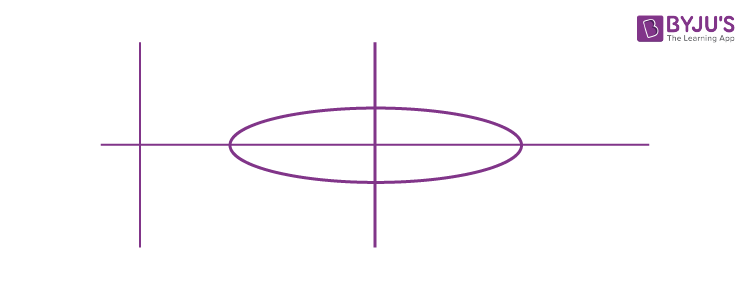x = –4

e = 1 / 2

(-a / e) = -4

-a = -4 * e

a = 2

Now, b2 = a2 (1 – e2) = 3

Equation to ellipse

(x2/4) + (y2/3) = 1

Equation of normal is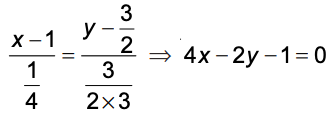Question 21: A hyperbola passes through the point P (√2, √3) and has foci at (±2, 0). Then the tangent to this hyperbola at P also passes through the point :

1) (2√2 , 3√3)
2) (√3, √2)
3) (− √2 , –√3)
4) (3√2 , 2√3)

[x2 / a2] – [y2 / b2] = 1

a2 + b2 = 4 and [2 / a2] – [3 / b2] = 1

(2 / [4 – b2]) – (3 / [b2]) = 1

b2 = 3

a2 = 1

x2 – (y2 / 3) = 1

∴ Tangent at P (√2 , √3) is √(2)x – (y / √3)) = 1

Clearly it passes through (2√2 , 3√3).

Question 22: The distance of the point (1, 3, −7) from the plane passing through the point (1, −1, −1), having normal perpendicular to both the lines

$$\begin{array}{l}\frac{x-1}{1}=\frac{y+2}{-2}=\frac{z-4}{3}\end{array}$$
and
$$\begin{array}{l}\frac{x-2}{2}=\frac{y+1}{-1}=\frac{z+7}{-1}\end{array}$$
is:

1) 10 / √83
2) 5 / √83
3) 10 / √74
4) 20 / √74

Let the plane be a (x – 1) + b (y + 1) + c (z + 1) = 0.

It is perpendicular to the given lines

a – 2b + 3c = 0

2a – b – c = 0

Solving, a : b : c = 5 : 7 : 3

The plane is 5x + 7y + 3z + 5 = 0

Distance of (1, 3, –7) from this plane = 10 / √83

Question 23: If the image of the point P (1, −2, 3) in the plane, 2x + 3y − 4z + 22 = 0 measured parallel to the line, (x / 1) = (y / 4) = (z / 5) is Q, then PQ is equal to :

1) 2√42
2) √42
3) 6√5
4) 3√5

Equation of PQ,

$$\begin{array}{l}\frac{x-1}{1}=\frac{y+2}{4}=\frac{z-3}{5}\end{array}$$

Let M be (λ + 1, 4λ – 2, 5λ + 3)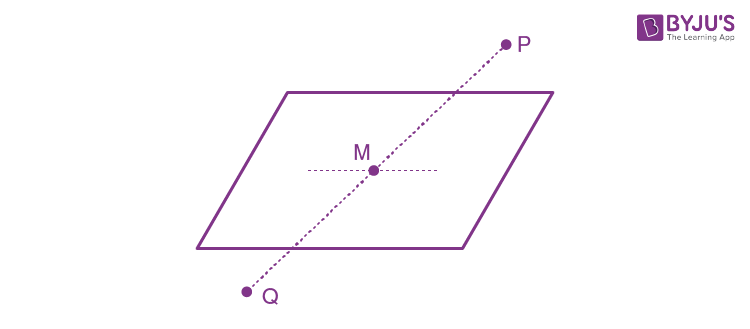As it lies on 2x + 3y – 4z + 22 = 0

λ = 1

For Q, λ = 2

Distance PQ = 2 √12 + 42 + 52 = 2√42

Question 24: Let a = 2i + j – 2k and b = i + j. Let c be a vector such that |c – a| = 3, |(a x b) x c| = 3 and the angle between c and a × b be 30o. Then a ⋅ c is equal to :

1) 2
2) 5
3) 1 / 8
4) 25 / 8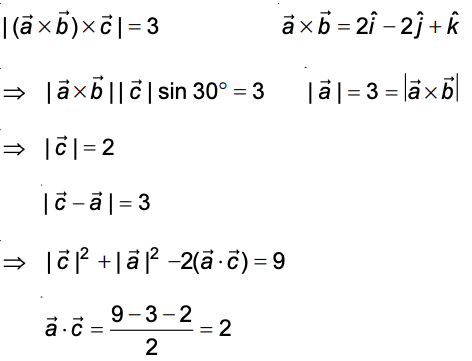Question 25: A box contains 15 green and 10 yellow balls. If 10 balls are randomly drawn, one-by-one, with replacement, then the variance of the number of green balls drawn is :

1) 6
2) 4
3) 6 / 25
4) 12 / 5

n = 10

p (Probability of drawing a green ball) = 15 / 25

p = 3 / 5, q = 2 / 5

var (X) = n.p.q

= 10 * (3 / 5) * (2 / 5)

= 10 * (6 / 25)

= 12 / 5

Question 26: For three events A, B and C, P (Exactly one of A or B occurs) = P (Exactly one of B or C occurs) = P (Exactly one of C or A occurs) = 1 / 4 and P (All the three events occur simultaneously) = 1 / 16. Then the probability that at least one of the events occurs is :

1) 7 / 16
2) 7 / 64
3) 3 / 16
4) 7 / 32

P (A) + P (B) – P (A ⋂ B) = 1 / 4

P (B) + P (C) – P (B ⋂ C) = 1 / 4

P (C) + P (A) – P (A ⋂ C) = 1 / 4

P (A) + P (B) + P (C) – P (A ⋂ B) – P (B ⋂ C) – P (A ⋂ C = 3 / 8

∵ P (A ⋂ B ⋂ C) = 1 / 16

∴ P (A ⋃ B ⋃ C) = (3 / 8) + (1 / 16) = 7 / 16

Question 27: If two different numbers are taken from the set {0, 1, 2, 3, ……, 10}; then the probability that their sum, as well as absolute difference, are both multiples of 4, is :

1) 12 / 55
2) 14 / 45
3) 7 / 55
4) 6 / 55

Total number of ways = 11C2 = 55

Favourable ways are (0, 4), (0, 8), (4, 8), (2, 6), (2, 10), (6, 10)

Probability = 6 / 55

Question 28: If 5(tan2 x − cos2 x) = 2cos2x + 9, then the value of cos 4x is :

1) 1 / 3
2) 2 / 9
3) −7 / 9
4) −3 / 5

5(tan2 x − cos2 x) = 2cos2x + 9

5 sec2 x – 5 = 9 cos2 x + 7

Let cos2 x = t

(5 / t) = 9t + 12

9t2 + 12t – 5 = 0

t = 1 / 3 as t ≠ – 5 / 3

cos2 x = 1 / 3, cos 2x = 2cos2 x – 1 = -1 / 3

cos 4x = 2cos2 2x – 1

= (2 / 9) – 1

= – 7/ 9

Question 29: Let a vertical tower AB have its end A on the level ground. Let C be the mid-point of AB and P be a point on the ground such that AP = 2AB. If ∠BPC = β, then tan β is equal to :

1) 1/4
2) 2/9
3) 4/9
4) 6/7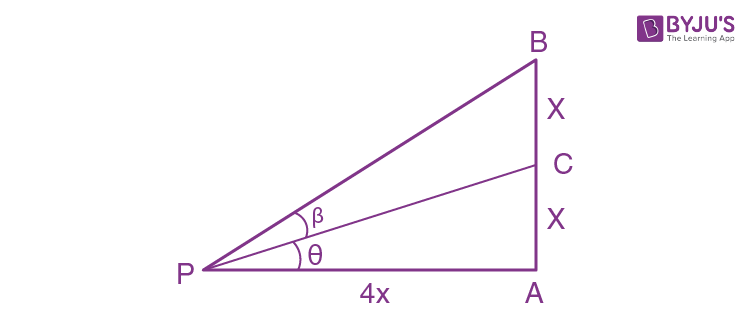tan θ = 1/4

tan (θ + β) = 1/2

$$\begin{array}{l}\frac{\frac{1}{4}+tan\beta}{1-\frac{1}{4}tan\beta}=\frac{1}{2}\\ tan\beta=\frac{2}{9}\end{array}$$

Question 30: The following statement (p → q) → [(~p → q) → q] is :

1) equivalent to ~p → q
2) equivalent to p → ~q
3) a fallacy
4) a tautology